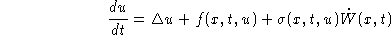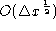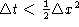# SCIENTIFIC PROGRAMS AND ACTIVITIESNovember 30, 2023## April 19 - 23, 1999 ABSTRACTS

### Monday, April 19, 1999Symposium on Numerical Stochastics in Finance

Phelim P. Boyle
Introduction to Modern Finance

In the the last 20 years there has been an explosive growth in financial innovation and the development of new financial markets. There are many reasons for this including advances in technology, deregulation and new breakthroughs in academic research. In this talk we discuss the basic ideas in modern finance staring with brief institutional details followed by a discussion of some of the key concepts. We will use a simple securities market model to illustrate the concepts of no arbitrage and incomplete markets and develop the connection between no arbitrage and the existence of an equivalent martingale measure. For the most part we will work in discrete time occasionally alluding to the continuous time formulation. We will contrast the no arbitrage approach with the equilibrium approach and discuss the valuation of new securities in an incomplete market. We will explore issue in valuation and hedging and indicate applications of the Monte Carlo method to exotic options and the estimation of portfolio risk. We will also discuss the famous (or infamous!) Value at Risk concept and mention some of the challenges it presents. We will mention the problem of valuation of American options in high dimensions and briefly outline the progress attained. The talk will assume little background by way of finance knowledge.

Pierre L'Ecuyer
Monte Carlo and Quasi-Monte Carlo Methods

The talk will discuss Monte Carlo and quasi-Monte carlo methods and give examples of their application in finance. Efficiency improvement techniques such as common random numbers, control variates, stratification, conditional Monte Carlo, and importance sampling, will be explained. The construction of random number generators, the philosophy behind, and their quality criteria will be discussed. Low-discrepancy point sets and sequences, such as lattice rules, nets, and randomized versions of them, will be covered. Concrete examples from finance, with numerical results, will be given.

Dietmar Leisen
Continuous-Time Finance and Its Approximations

This talk discusses pricing and hedging in continuous time and practical implementations to calculate prices in asset market models. We start with a financial model where prices follow geometric Brownian motion (Black-Scholes model). We discuss Girsanov's theorem, the Feynman-Kac representation of prices as expectations and the Black-Scholes PDE. We do then discuss lattice approximations and the specific numerical difficulties, which arise: convergence issues, order of convergence, and possible improvements. We also study incomplete markets and generalize the model in several directions: one-dimensional diffusions, multinomial diffusions, jump-diffusions and stochastic volatility models.
Philip Protter
Numerical Methods for Stochastic Differential Equations arising in Finance
A standard problem in Finance Theory is to price derivatives and to estimate hedging strategies. The simple Black-Scholes paradigm gives rise often to formulas which are explicit or involve solutions of PDEs. If one leaves the realm of modeling security prices with linear SDEs, however, and uses non linear coefficients, then the usual methods break down, and one must rely on simulations of the solutions of SDEs combined with Monte Carlo techniques. We will focus on the problem of pricing of derivatives, however the hedging problems can be handled similarly. We discuss recent attempts to mathematically analyze this type of procedure: for example if we use an Euler scheme for the SDE and combine it with a Monte Carlo scheme, what is the error? How should the step size of the Euler scheme be related to the number of simulation? We then discuss the situation when the paradigm is further generalized to include jumps. This leads to complete models through the Azema martingales, and to incomplete models via (eg) Levy differentials. In the latter case the mathematical analysis is just beginning and some simulation problems arise.

### Tuesday, April 20, 1999 - Friday, April 23, 1999Workshop on Numerical Methods and Stochastics

Dan Crisan
Numerical methods for solving the stochastic filtering problem
We present a survey of existing approaches to solve numerically the stochastic filtering problem: linearisation methods (extended Kalman filter), approximation by finite dimensional non-linear filters, particle filters, classical partial differential equations methods, Wiener Chaos expansions, moment methods. We explain some of the differences between these methods and make a few comparative remarks.
Optimal filtering on binary trees
The stochastic filtering problem is stated for signal processes evolving on discrete sets, in particular on binary trees. Approximating algorithms for generating the posterior distribution of the signal are introduced and optimality results regarding the mean relative entropy of the approximating measure with respect to the posterior measure are presented.
Pierre Del Moral
Genetic/particle algorithms and their application to integration of functionals in high dimensions

The talk will survey the applications of methods arising in the study of interacting particly systems, and in genetic models, to problems of approximating various high-dimensional integrals.
Branching and Interacting Particle Systems Approximations of Feynman-Kac Formulae with Applications to Non Linear Filtering
This talk focuses on interacting particle system methods for the numerical solving of a class of Feynman-Kac formulae arising in the study of certain parabolic differential equations, physics, non linear filtering and elsewhere. We will give an expos\'e of the mathematical theory that may be useful in analyzing the convergence of such particle approximating models including law of large numbers, large deviations principles, fluctuations and empirical process theory as well as semi-group techniques and limit theorems for processes.
In addition, we will investigate the delicate and probably the most important problem of the long time behavior of such interacting measure valued processes. We will show how to connect this problem with the asymptotic stability of the corresponding limiting process so that to derive useful uniform convergence results with respect to the time parameter.

Jessica Gaines
Discretisation methods for numerical solution of SDE's and SPDE'S

After a brief reminder of the use of discretization schemes for approximation of (mainly pathwise) solutions of stochastic differential equations, the talk will review work to date on numerical solution of stcohastic partial differential equations (SPDE's). Both finite difference and finite element methods will be covered. Most work has been done on parabolic equations, but there are also some initial results and/or experiments involving the solution of elliptic and hyperbolic SPDE's.

Optimal convergence rates of discretisation methods for parabolic SPDE's

We answer the question "What is the best rate of convergence obtainable when solving the parabolic SPDEusing a finite difference method in a certain class?" Gyöngy showed that the most obvious method has rate of convergence  with  . Can one do better than this? If so, how?

Alice Guionnet
Particle systems approximations of non-linear differential equations I
Particle systems approximations of non-linear differential equations II

We shall review some examples where particle methods are used to approximate physical quantities, such as solutions to non-linear differential equations of non-linear stochastic differential equations. We shall analyze convergence, large deviations, and central limit theorems for such approximations. Applications to non-linear filtering problems will also be given.

Terry Lyons
Mathematical problems in numerical stochastic analysis

This talk is intended to be informal, and to set out a series of questions and connections in the scientific computation of stochastic systems. The aim is to expose problems where mathematical progress would yield benefit.
Rough paths and variable steps in the numerical analysis of fractional and classical stochastic processes
Rough Paths give a new way of approximating the underlying noise in many stochastic systens. This can be exploited in new algorithms.

Laurent Miclo
Time-continuous interacting particle approximations of Feynman-Kac formulae

We will present a weighted sampling Moran particle system model for the numerical solving of the Feynman-Kac formulae appearing, among others, in the non-linear filtering equations. Up to some classical transformations, the latter can also be seen as a simple (but time-inhomogeneous and in a random environment given by the observations) generalized spatially homogeneous Boltzmann equation, so our general continuous time approximation is also related to the corresponding Nanbu-type interacting particle systems. But we will develop a new proof rather based on martingales and semigroup techniques to prove the convergence when the number of particles increases with an upper bound on the speed of convergence which is typical of the propagation of chaos. We also establish a functional central limit theorem for the fluctuations, and under some mixing assumptions, we will get uniform convergence results with respect to the time parameter.

Philip Protter
Some recent results concerning numerical methods for stochastic differential equations

We will survey some numerical techniques used to analyze stochastic differential equations, and recent theorems concerning the mathematical analysis of such techniques. For example if one uses an Euler scheme to approximate a solution of an SDE, one can describe the asymptotic normalized error. Then if one adds a Monte Carlo approximation to evaluate a function of the solution, one can analyze the asymptotic normalized error of the entire solution. This reveals the interplay between the number of simulations and the partition step size. We will then discuss new directions of research for numerical methods: non Brownian models such as SDEs driven by Levy processes; functionals of the paths of the solutions instead of simply functions of the paths; forward-backward equations; and backward equations. This is a research area in its infancy.

Frederi Viens
Simulating the fast dynamo problem for stochastic magneto-hydrodynamics via discretized Feynman-Kac formulae

In a viscous magnetic fluid with given velocity field, the magnetic field H is a 3d-vector-valued random field on(or a domain of) which satisfies a linear system of parabolic partial differential equations. If the velocity field is assumed to be white-noise in time and smooth in space, then H will satisfy a linear system of three stochastic PDEs, in which the zero- and first-order terms have coefficients that are white-noise in time. It is conjectured that the exponential rate of change of the norm of H for large time is constant (almost-sure Lyapunov exponent) and, as the viscosity increases from zero to a positive value, this rate of change increases very fast (fast dynamo random generation). An explicit Feynman-Kac-type formula for H is available, which can be discretized in time and space, offering theoretical as well as numerical possibilities for tackling this difficult problem, including using the theory of products of random matrices, and simulations by particle techniques.

John Walsh
Stochastic Partial Differential Equations Brownian motion and convergence rates of binomial tree methods

Abstracts not yet available